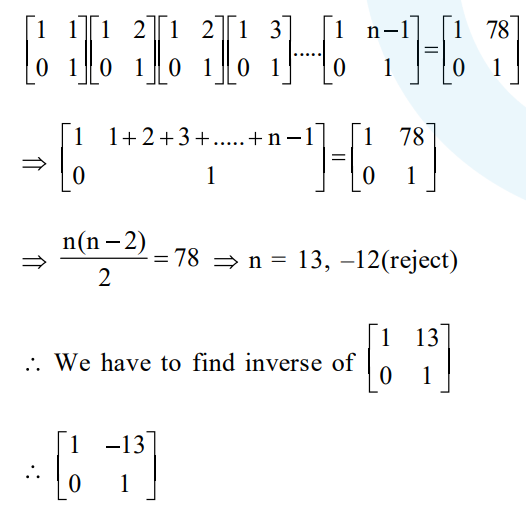# Solve this following

Question:

If $\left[\begin{array}{ll}1 & 1 \\ 0 & 1\end{array}\right] \cdot\left[\begin{array}{ll}1 & 2 \\ 0 & 1\end{array}\right] \cdot\left[\begin{array}{ll}1 & 3 \\ 0 & 1\end{array}\right] \ldots . .\left[\begin{array}{cc}1 & \mathrm{n}-1 \\ 0 & 1\end{array}\right]=\left[\begin{array}{cc}1 & 78 \\ 0 & 1\end{array}\right]$, then

the inverse of $\left[\begin{array}{ll}1 & \mathrm{n} \\ 0 & 1\end{array}\right]$ is

1. $\left[\begin{array}{cc}1 & -13 \\ 0 & 1\end{array}\right]$

2. $\left[\begin{array}{cc}1 & 0 \\ 12 & 1\end{array}\right]$

3. $\left[\begin{array}{cc}1 & -12 \\ 0 & 1\end{array}\right]$

4. $\left[\begin{array}{cc}1 & 0 \\ 13 & 1\end{array}\right]$

Correct Option: 1

Solution: